Oct. 5, 2019

# Medical Images In python (Computed Tomography)

This post aims to provide the basic knowledge of medical images and how to load and process these images to use them to train deep learning models, I am not an expert, therefore some of this information is basic but usefully.

In this post I will use python to load and visualize the images, additionally, all the images are from the brain but you can apply a lot of these concepts for images of other organs.

This is the first part of two posts where we will talk about CT Images, in the next post we will talk about MRI and PET images. Even if you are only interested in MRI and PET images you should read this post as well since there are a lot of useful information.

## Pydicom and NiBabel

Normally in datasets like ImageNet images have the jpg format. However, in medical stuff, DICOM and NIfTI formats are used. The main difference between these two formats is that the DICOM format has multiple 2d image slices which together form a 3d image, whereas the NIfTI format has only one file that contains the 3d image. We can use a library called Pydicom to open DICOM images and NiBabel to open NIfTI images. Due to the fact that both libraries load the images in a numpy array, it's easy to get and save 2d images in the jpg format. In the following sections, we will use these libraries to open different types of medical images.

## Anatomical Planes

Since the images we are working with are 3d images, we can get multiple views and obtain different information from each one. These views are called anatomical planes: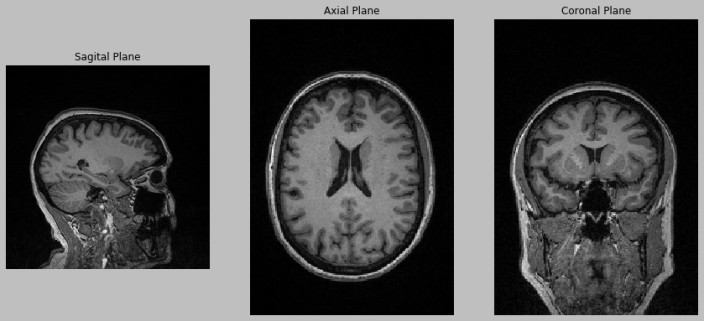In the image above we can see the three anatomical planes.

In this post, we will work mainly on the axial plane. In fact, switch between one plane and another is quite easy

``````
import nibabel as nib

import numpy as np

import matplotlib.pyplot as plt

def display_views(file_path):

image_axis = 2

image = medical_image.get_data()

sagital_image = image[110, :, :] # Axis 0

axial_image = image[:, :, 144] # Axis 2

coronal_image = image[:, 144, :] # Axis 1

plt.figure(figsize=(20, 10))

plt.style.use('grayscale')

plt.subplot(141)

plt.imshow(np.rot90(sagital_image))

plt.title('Sagital Plane')

plt.axis('off')

plt.subplot(142)

plt.imshow(np.rot90(axial_image))

plt.title('Axial Plane')

plt.axis('off')

plt.subplot(143)

plt.imshow(np.rot90(coronal_image))

plt.title('Coronal Plane')

plt.axis('off')

``````

In the code above we used NiBabel to load a NIfTI image and matplotlib to plot the image planes, we only have to change the axis used in the numpy array.

## Computed Tomography (CT Images)

Computed Tomography scans produce images of the body using X-rays. We can use a head CT to evaluate various structures of the brain and look for abnormalities, areas of bleeding, stroke, where the brain's blood supply is inadequate, among other things.

The unit of measurement in CT scans is the Hounsfield Unit (HU) which is a measure of radiodensity. Each voxel (3D pixel) of a CT scan has an attenuation value that is the measure of the reduction intensity of a ray of light by the tissue through it passes.

Each pixel is assigned to a numerical value called CT Number, which is the average of all the attenuation values contained within the corresponding voxel. This CT Number is compared to the attenuation value of the water and displayed on a scale named Hounsfield units. On this scale, the water has an attenuation value of zero and the range of CT numbers could be up to 4000. Each number represents a shade of grey, this means that we could have up to 4000 shades of grey.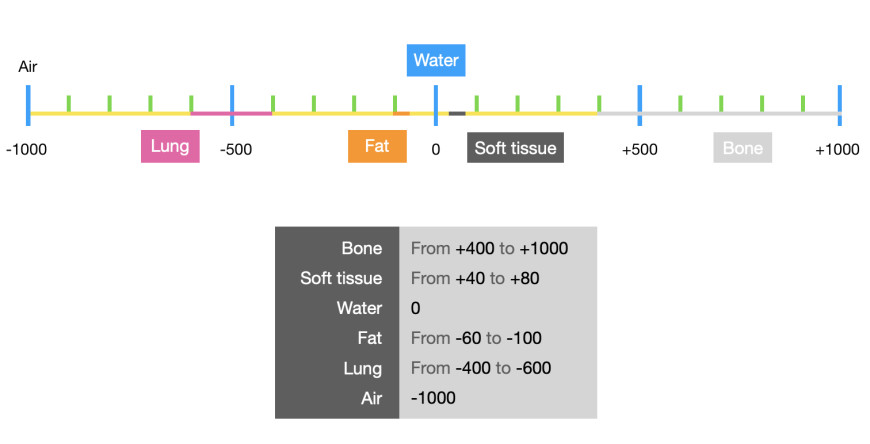In this scale the +1000 values are completely white like the 255 value in an RGB image and the -1000 values are completely black like the 0 value in an RGB image

### Windowing

Since neither we nor the machines can recognize 4000 shades of gray easily, we use a technique called windowing to limit the number of Hounsfield units that are displayed. For example if we want to examine the soft tissue in one CT scan we can use a window level of 40 and a window Width of 80 this will cover 40 units below and above the window level and the tissues with CT numbers outside this range will appear either black or white. A narrow range provides a higher contrast.

### Pydicom

Now we will load a DICOM image of the brain and display different scales using different values for window level and window width.

Additionally we must know that this kind of images contain meta information that is sensitive like information about the patient.Therefore, we should take this into account and be carefully.

With the code below we can load a DICOM image using pydicom:

``````
import pydicom

import numpy as np

import cv2

import os

import matplotlib.pyplot as plt

file_path = "ID_000039fa0.dcm"

output_path = "./"

``````

We can print the medical_image variable and see the headers of the file:

``````
print(medical_image)

``````
``````
(0028, 0010) Rows                                US: 512

(0028, 0011) Columns                             US: 512

(0028, 0030) Pixel Spacing                       DS: ['0.488281', '0.488281']

(0028, 0100) Bits Allocated                      US: 16

(0028, 0101) Bits Stored                         US: 16

(0028, 0102) High Bit                            US: 15

(0028, 0103) Pixel Representation                US: 1

(0028, 1050) Window Center                       DS: "30"

(0028, 1051) Window Width                        DS: "80"

(0028, 1052) Rescale Intercept                   DS: "-1024"

(0028, 1053) Rescale Slope                       DS: "1"

``````

To get the pixel data from the image we use the following code:

``````
image = medical_image.pixel_array

``````

We can also get the size of the slice:

``````
print(image.shape)

``````
``````
(512, 512)

``````

We can notice that the image only have two dimensions since the DICOM files only contains one slice per file.

Now we can print the range of values of the image:

``````
print(image.min())

print(image.max())

``````
``````
-2000

2848

``````

Now we have to transform the pixel values to the Hounsfield units, we can achieve this using the headers in the medical_image file, we will use the Rescale Intercept and Rescale Slope headers:

``````
def transform_to_hu(medical_image, image):

intercept = medical_image.RescaleIntercept

slope = medical_image.RescaleSlope

hu_image = image * slope + intercept

return hu_image

``````

If we want a specific zone of the image we can windowing the image:

``````
def window_image(image, window_center, window_width):

img_min = window_center - window_width // 2

img_max = window_center + window_width // 2

window_image = image.copy()

window_image[window_image < img_min] = img_min

window_image[window_image > img_max] = img_max

return window_image

``````

Here we can see the the image in Hounsfield units and two windowing images, the first soft tissue and the second the bones

``````
hu_image = transform_to_hu(image)

brain_image = window_image(hu_image, 40, 80)

bone_image = window_image(hu_image, 400, 1000)

``````

We can load multiple images and apply this preprocess: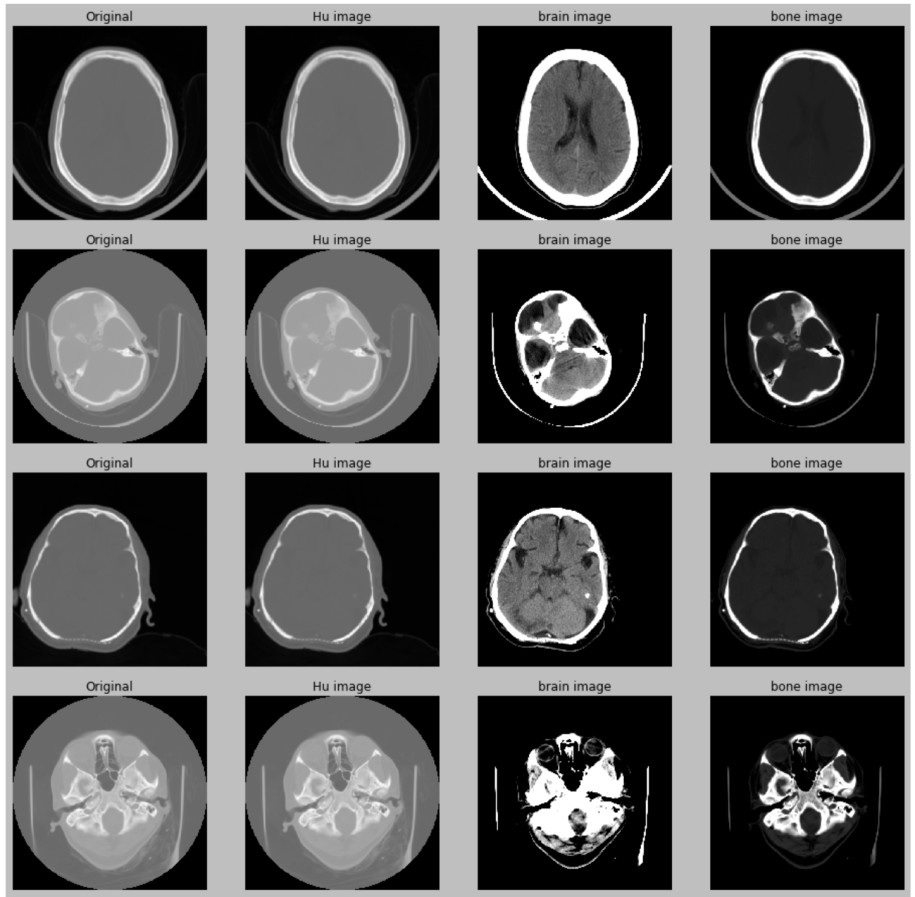I plotted these images using matplotlib. Since matplotlib can handle images in float32 format we can appreciate multiple shades of grey, if you want to save these images with the same results you should use mpimg.imsave, instead if you use a function like cv2.imwrite which only handles the uint8 format the final image will lose a lot of information and we won't be able to see them correctly. We can also use np.save to save the array/image to a binary file in the .npy format.

### Improving the images

We have seen how we can load CT images, transform to Hounsfield units, windowing and plot them. However, we can improve the quality of these images by removing noise and adjusting their size:

``````
def remove_noise(file_path, display=False):

image = medical_image.pixel_array

hu_image = transform_to_hu(medical_image, image)

brain_image = window_image(hu_image, 40, 80)

# morphology.dilation creates a segmentation of the image

# If one pixel is between the origin and the edge of a square of size

# 5x5, the pixel belongs to the same class

# We can instead use a circule using: morphology.disk(2)

# In this case the pixel belongs to the same class if it's between the origin

# and the radius

segmentation = morphology.dilation(brain_image, np.ones((5, 5)))

labels, label_nb = ndimage.label(segmentation)

label_count = np.bincount(labels.ravel().astype(np.int))

# The size of label_count is the number of classes/segmentations found

# We don't use the first class since it's the background

label_count = 0

# We create a mask with the class with more pixels

# In this case should be the brain

mask = labels == label_count.argmax()

# Improve the brain mask

# Since the the pixels in the mask are zero's and one's

# We can multiple the original image to only keep the brain region

if display:

plt.figure(figsize=(15, 2.5))

plt.subplot(141)

plt.imshow(brain_image)

plt.title('Original Image')

plt.axis('off')

plt.subplot(142)

plt.axis('off')

plt.subplot(143)

plt.title('Final Image')

plt.axis('off')

``````

With the code above we can remove the noise from the outside of the brain: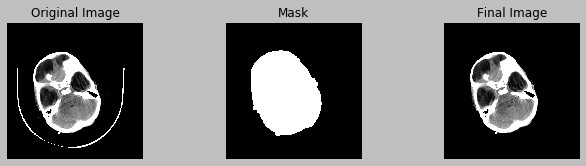Not all the images are centered, we can also center the images and change their size:

``````
def crop_image(image, display=False):

# Create a mask with the background pixels

mask = image == 0

# Find the brain area

top_left = np.min(coords, axis=1)

bottom_right = np.max(coords, axis=1)

# Remove the background

croped_image = image[top_left:bottom_right,

top_left:bottom_right]

return croped_image

height, width = image.shape

final_image = np.zeros((new_height, new_width))

pad_left = int((new_width - width) / 2)

pad_top = int((new_height - height) / 2)

# Replace the pixels with the image's pixels

return final_image

``````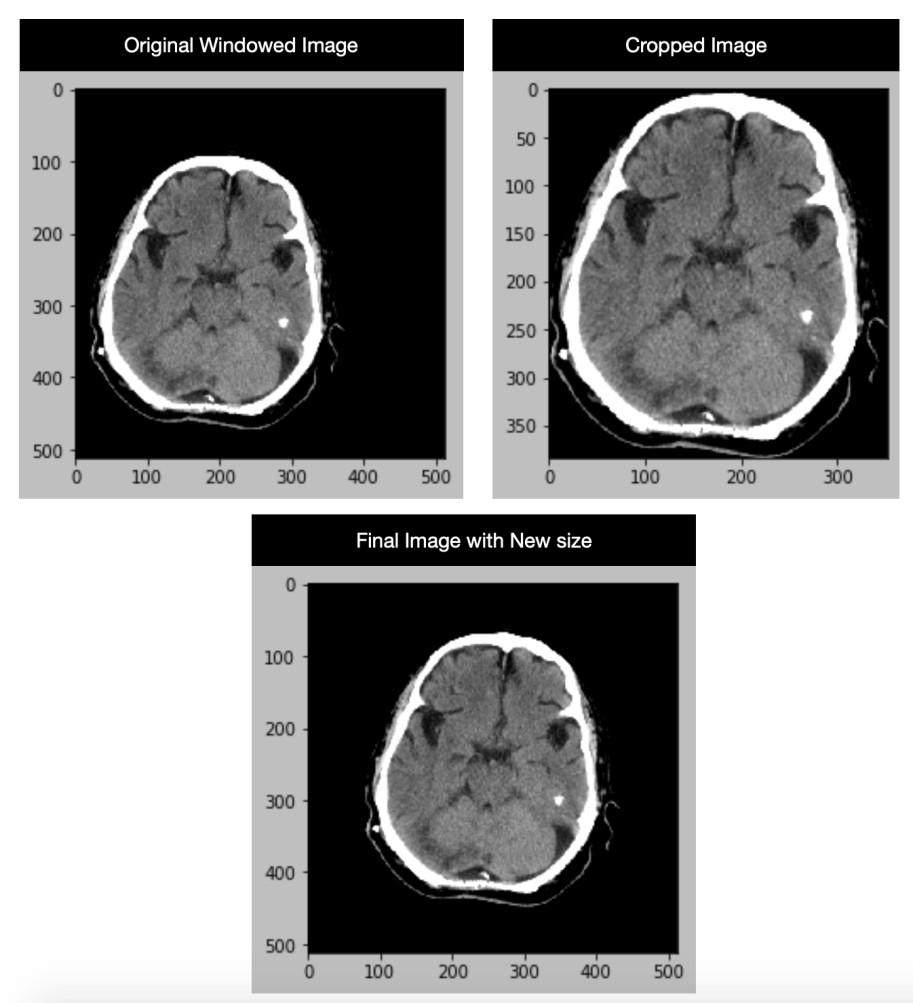One thing I would like to mention is that if we save the images with mpimg.imsave and load these images with the same function the range of the images will be [0-1]. However, if we use cv2.imwrite to load these images the range will be [0-255].

## Resampling

Medical images don't have a standard size but we can fix that and use a size like 1x1x1mm³. Firstly, we will look at the thickness/width and at the size of each voxel:

``````

# we use the last axis

image_thickness = np.abs(first_medical_image.ImagePositionPatient - second_medical_image.ImagePositionPatient)

pixel_spacing = first_medical_image.PixelSpacing

``````
``````
image_thickness = 0.6430000000000007

pixel_spacing = ['0.501953', '0.501953']

``````

We need two slices to compute the distance between them, we can see that the thickness/width of each slice is 0.6430000000000007mm and the size of each voxel is 0.501953mm. Thus, the size of each slice is 0.643x0.501953x0.501953mm³

Now we have to change the size of each slice to 1x1x1mm³:

``````
def resample(image, image_thickness, pixel_spacing):

new_size = [1, 1, 1]

x_pixel = float(pixel_spacing)

y_pixel = float(pixel_spacing)

size = np.array([x_pixel, y_pixel, float(image_thickness)])

image_shape = np.array([image.shape, image.shape, 1])

new_shape = image_shape * size

new_shape = np.round(new_shape)

resize_factor = new_shape / image_shape

resampled_image = ndimage.interpolation.zoom(np.expand_dims(image, axis=2), resize_factor)

return resampled_image

``````
``````
first_image = first_medical_image.pixel_array

first_image.shape

``````
``````
(512, 512)

``````
``````
resampled_image = resample(first_image, image_thickness, pixel_spacing)

np.squeeze(resampled_image).shape

``````
``````
(257, 257)

``````

As we can see, the size of the final image changed since each voxel represented 0.501953mm and now each voxel represents 1mm.

With this technique, a neural network could improve the predictions in different images since they will have the same spatial information and the kernels can learn this information in the same way.

You can see a jupyter notebook with all the code of this section in this link.